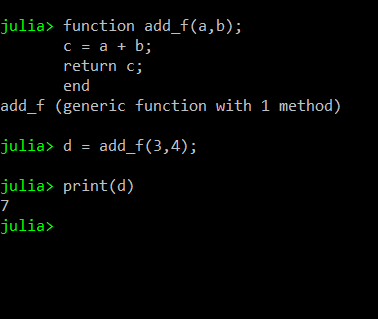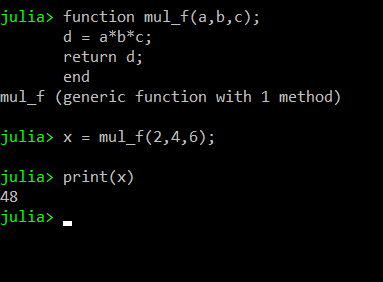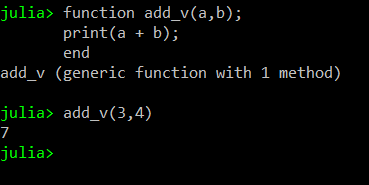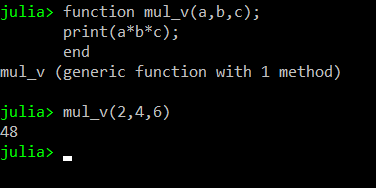Related Articles
Fruitful Functions and Void Functions in Julia
• Last Updated : 25 Aug, 2020

Functions are one of the most useful tools when writing a program. Every programming language including Julia uses functions, it can be for keeping the code simple and readable or to keep the program secure from outside interference. In almost every programming language there are two types of functions:

• Fruitful Functions
• Void Functions

#### Fruitful Functions

These are the functions that return a value after their completion. A fruitful function must always return a value to where it is called from. A fruitful function can return any type of value may it be string, integer, boolean, etc. It is not necessary for a fruitful function to return the value of one variable, the value to be returned can be an array or a vector. A fruitful function can also return multiple values.

Example 1:

 `# Creation of Function ` `function add_f(a, b); ` `    ``c ``=` `a ``+` `b; ` `     `  `    ``# Returning the value ` `    ``return` `c; ` `end ` ` `  `# Function call ` `d ``=` `add_f(``3``, ``4``); ` `print``(d) `

Output:Example 2:

 `# Creation of Function ` `function mul_f(a, b, c); ` `    ``d ``=` `a ``*` `b ``*` `c; ` `     `  `    ``# Returning the result  ` `    ``# to caller function ` `    ``return` `d; ` `end ` ` `  `# Function call ` `x ``=` `mul_f(``2``, ``4``, ``6``); ` `print``(x) `

Output:#### Void Functions

Void functions are those that do not return any value after their calculation is complete. These types of functions are used when the calculations of the functions are not needed in the actual program. These types of functions perform tasks that are crucial to successfully run the program but do not give a specific value to be used in the program.

Example 1:

 `# Creation of Function ` `function add_v(a, b); ` `    ``c ``=` `a ``+` `b; ` `    ``print``(c); ` `end ` ` `  `# Function Call ` `add_v(``3``, ``4``) `

Output:Example 2:

 `# Creation of Function ` `function mul_v(a, b, c) ` `    ``print``(a ``*` `b ``*` `c); ` `end ` ` `  `# Function Call ` `mul_v(``2``, ``4``, ``6``) `

Output:My Personal Notes arrow_drop_up
Recommended Articles
Page :